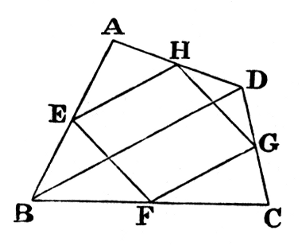#Pillow-Problems: Problem #3

Math Lair Home > Source Material > Pillow-Problems > Problem #3

## Problem:

3. (30)

If the sides of a Tetragon pass through the vertices of a Parallelogram, and if three of them are bisected at those vertices: prove that the fourth is so also.

## Solution:

3.Let ABCD be the Tetragon; and let the 3 sides, AB, BC, CD, be bisected by vertices of the Parallelogram EFGH.

Join BD.

∵ in Triangle BCD, sides BC, CD are bisected at F and G,

FG is parallel to BD;

but EH is parallel to FG;

EH is parallel to BD;

∴ Triangles AEH, ABD are similar;

now AE is half of AB;

AH is half of AD.

Q.E.D.